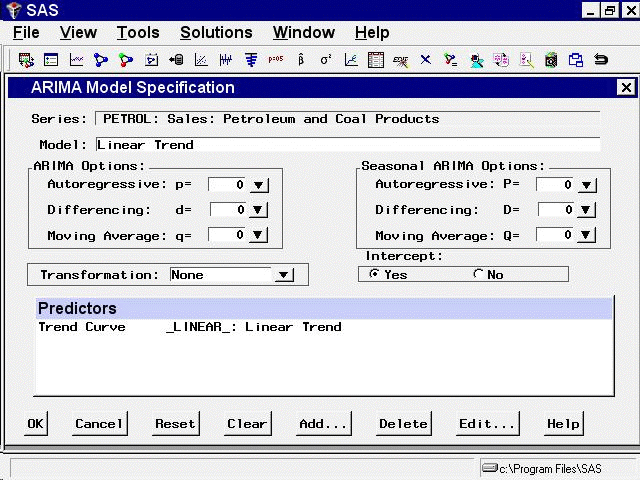# Using Predictor Variables

## Linear Trend

From the Develop Models window, select `Fit ARIMA Model.` From the ARIMA Model Specification window, select `Add` and then select `Linear Trend` from the menu (shown in Figure 50.1).

A linear trend is added to the Predictors list, as shown in Figure 50.3.

Figure 50.3: Linear Trend Predictor SpecifiedThe description for the linear trend item shown in the Predictors list has the following meaning. The first part of the description, Trend Curve, describes the type of predictor. The second part, _LINEAR_, gives the variable name of the predictor series. In this case, the variable is a time index that the system computes. This variable is included in the output forecast data set. The final part, Linear Trend, describes the predictor.

Notice that the model you have specified consists only of the time index regressor _LINEAR_ and an intercept. Although this window is normally used to specify ARIMA models, in this case no ARIMA model options are specified, and the model is a simple regression on time.

Select the `OK` button. The Linear Trend model is fit and added to the model list in the Develop Models window.

Now open the Model Viewer by using the `View Model Graphically` icon or the `Model Predictions` item under the `View` pull-down menu or toolbar. This displays a plot of the model predictions and actual series values, as shown in Figure 50.4. The predicted values lie along the least squares trend line.

Figure 50.4: Linear Trend Model